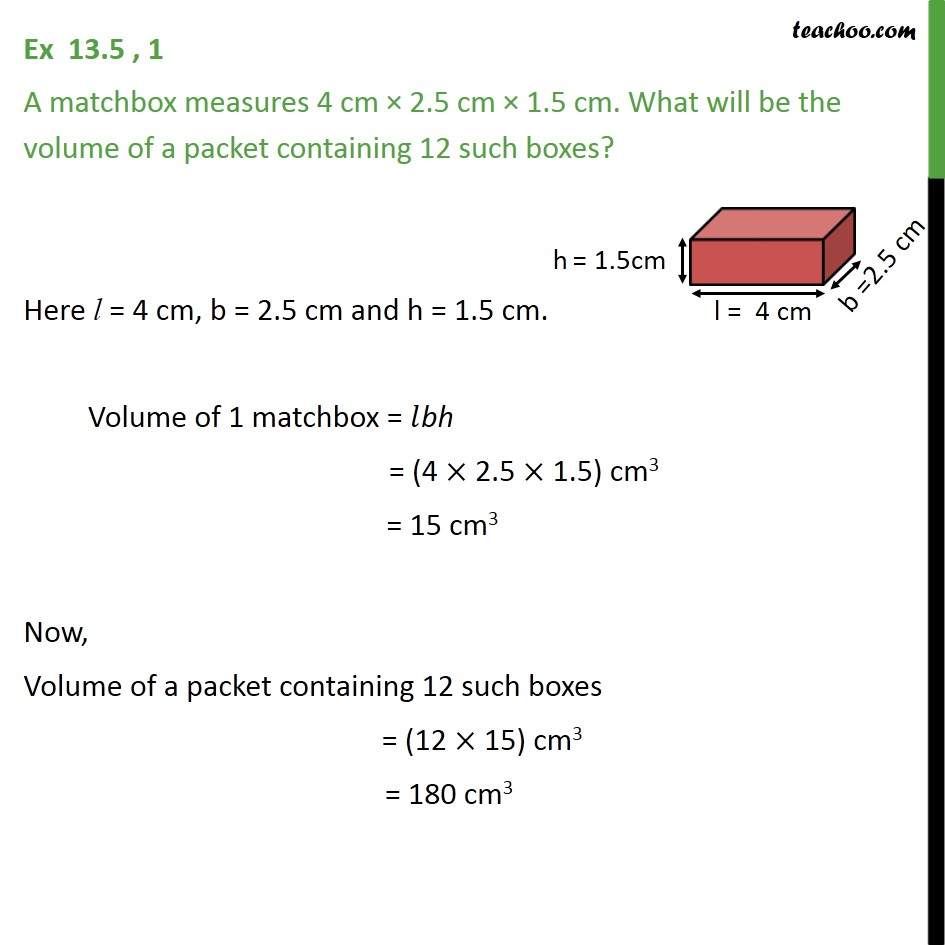1. Chapter 13 Class 9 Surface Areas and Volumes (Term 2)
2. Serial order wise
3. Ex 13.5

Transcript

Ex 13.5 , 1 A matchbox measures 4 cm 2.5 cm 1.5 cm. What will be the volume of a packet containing 12 such boxes? Here l = 4 cm, b = 2.5 cm and h = 1.5 cm. Volume of 1 matchbox = bh = (4 2.5 1.5) cm3 = 15 cm3 Now, Volume of a packet containing 12 such boxes = (12 15) cm3 = 180 cm3